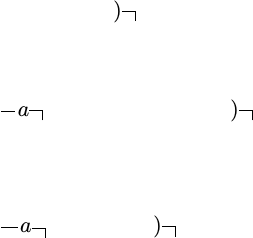Study Guides (380,000)
CA (150,000)
UW (6,000)
ACTSC (100)
Quiz

# ACTSC231 Study Guide - Quiz Guide: Effective Interest Rate, Problem Set, Royal Institute Of TechnologyExam

Department
Actuarial Science
Course Code
ACTSC231
Professor
Chengguo Weng
Study Guide
Quiz

This preview shows half of the first page. to view the full 3 pages of the document.Actsc 231 - Problem Set 7
This problem set is composed mainly of recommended problems from the course textbook.
For the textbook problems, at the beginning of the question, a reference will be made to the
exercise from which it was taken.
1. Find the present value of a 10-year continuously paying annuity if the payment rate at
time tis t5and the force of interest is 0.002t,0t10.
2. Surprise! You’re back in the Den and Kevin wants to invest money today in your busi-
ness. In exchange, he wants a royalty on your sales of \$1 per unit sold until the \$10,000
is repaid (so in this case, you need to sell 10,000 units) and then a royalty of \$0.50 per
unit sold thereafter (forever). Assume money is worth i(1) = 5%.
(a) Assume that you will sell 1,000 units each year forever. Assuming that the royalties
will be paid at the end of each year, what is the minimum investment you will ac-
cept from Kevin?
(b) Assume that you will sell 1,000 units each year forever. Assuming that the royalties
will be paid continuously throughout each year, what is the minimum investment
you will accept from Kevin?
(c) Assume that you will sell 1,000 units in the ﬁrst year, 2,000 in the second year, 3,000
in the third year, . . . ,10,000 in the tenth year and 0 units thereafter. Assuming that
the royalties will be paid at the end of the year, what is the minimum investment
you will accept from Kevin?
(d) Assume that you will sell 1,000 units in the ﬁrst year, 2,000 in the second year, 3,000
in the third year, . . . ,10,000 in the tenth year and 0 units thereafter. Assuming that
the royalties will be paid continuously throughout each year, what is the minimum
investment you will accept from Kevin?
3. Find the present value, (¯
D¯a)n i of an annuity which pays continuously at an annual rate
of ntat time tfor the next nyears. The annual effective interest rate is i.
4. (a) Show that d
di an i =(1 + i)1·(Ia)n i.
(b) Let’s treat the effective annual interest rate ias a function of δ; we will write i(δ)to
make this understood (that it is a function of δ). We know that i(δ) := i=eδ1.
Show that d
an i(δ)=(Ia)n i.
5. A loan is repaid by yearly payments of \$100, \$150, \$200, . . . , \$500. Assume payments are
made at the end of the year, with the ﬁrst payment being made 1 year after the loan is
taken out. Determine the total interest paid using an annual effective rate of 5%.
1